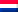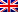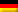Select Page

# When to replace the Audi A3 timing belt

The Audi A3 is a compact mid-size model from Audi. This model comes with both petrol and diesel engines. Below is an overview of the timing belt replacement intervals. If you don't know which engine your car has, you can enter the license plate number below.

## Petrolvariants:

• Audi A3 1.2 TSI (from 2010) = 1.0 Timing chain;
• Audi A3 1.2 TSI (from 2013) = 1.0 210,000 Kilometres;
• Audi A3 1.2 TFSI (from 2014) = 1.0210,000 Kilometres;
• Audi A3 1.4 TFSI (period 2007 - 2012) = Timing chain;
• Audi A3 1.4 TFSI (from 2012) = 1.0210,000 Kilometres;
• Audi A3 1.6 = 120 000 Kilometres or up to 5 years;
• Audi A3 1.6 FSI = Timing chain;
• Audi A3 1.8 = 180 000 Kilometres or up to 5 years;
• Audi A3 1.8 TFSI =Timing chain;
• Audi A3 1.8 TFSI quattro =Timing chain;
• Audi A3 1.8 T = 180 000 Kilometres or up to 5 years;
• Audi A3 1.8 T quattro = 180 000 Kilometres or up to 5 years;
• Audi A3 2.0 FSI = 180 000 Kilometre;
• Audi A3 2.0 TFSI = 180 000 Kilometre;
• Audi A3 2.0 TFSI (CAWB) = Timing chain;
• Audi A3 2.0 TFSI quattro = 180 000 Kilometre;
• Audi A3 2.0 TFSI quattro (CCZA) = Timing chain;
• Audi A3 S3 quattro (up to 2012) = 180 000 Kilometres or up to 5 years;
• Audi A3 S3 quattro (from 2012) =Timing chain;
• Audi A3 S3 quattro (AMK) = 120 000 Kilometre;
• Audi A3 3.2 V6 quattro = Timing chain.

## Diesel variants:

• Audi A3 1.6 TDI (period 2009 - 2012) =210 000 Kilometre;
• Audi A3 1.6 TDI (from 2012) = 1.0210 000 Kilometre;
• Audi A3 1.9 TDI (up to 1999) =60 000 Kilometre;
• Audi A3 1.9 TDI (2000 onwards) =90 000 Kilometre;
• Audi A3 1.9 TDI (from 2002) =120 000 Kilometre;
• Audi A3 1.9 TDI (from 2006) =120 000 Kilometre;
• Audi A3 1.9 TDI (from 2009) =150 000 Kilometre;
• Audi A3 1.9 TDI AZV (up to 2006) =120 000 Kilometre;
• Audi A3 1.9 TDI AZV (from 2007) =150 000 Kilometre;
• Audi A3 1.9 TDI CBAA (up to 2009) =180 000 Kilometre;
• Audi A3 1.9 TDI CBAA (from 2010) = 1.0210 000 Kilometre;
• Audi A3 2.0 TDI (up to 2006) =120 000 Kilometre;
• Audi A3 2.0 TDI (period 2007 - 2012) =150 000 Kilometre;
• Audi A3 2.0 TDI (from 2012) =210 000 Kilometre;
• Audi A3 2.0 TDI quattro (from 2012) =210 000 Kilometre;
• Audi A3 2.0 TDI BMN (up to 2006) =120 000 Kilometre;
• Audi A3 2.0 TDI BMN (from 2007) =150 000 Kilometre;
• Audi A3 2.0 TDI CBBB (up to 2009) =180 000 Kilometre;
• Audi A3 2.0 TDI CBBB (from 2010) =210 000 Kilometre;
• Audi A3 2.0 TDI CFGB =210 000 Kilometre;
• Audi A3 2.0 TDI 16V BKD (up to 2006) =120 000 Kilometre;
• Audi A3 2.0 TDI 16V BKD (from 2007) =150 000 Kilometre;
• Audi A3 2.0 TDI 16V CBAB (up to 2009) =180 000 Kilometre;
• Audi A3 2.0 TDI 16V CBAB (from 2010) = 1.0210 000 Kilometre;
• Audi A3 2.0 TDI 16V CFFB =210 000 Kilometre;
• Audi A3 2.0 TDI 16V quattro BKD (up to 2006) =120 000 Kilometre;
• Audi A3 2.0 TDI 16V quattro BKD (from 2007) = 1.0150 000 Kilometre;
• Audi A3 2.0 TDI 16V quattro CBAB (up to 2009) =180 000 Kilometre;
• Audi A3 2.0 TDI 16V quattro CBAB (from 2010) = 1.0210 000 Kilometre;
• Audi A3 2.0 TDI 16V quattro CFFB =210 000 Kilometre.NLNLENDE